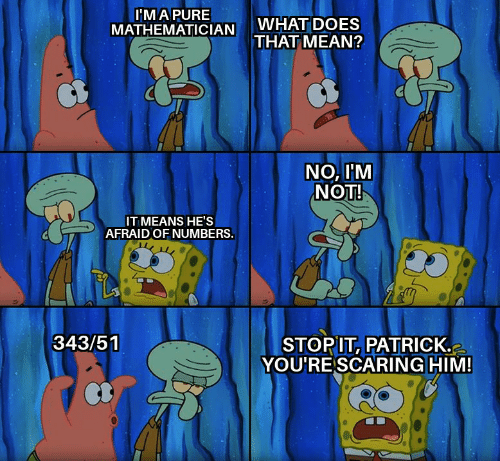### Papers

1. On smoothing singularities of elliptic orbital integrals on GL(n) and Beyond Endoscopy, with O.E. Gonzalez, C.H. Kwan, S.J. Miller, R. Van Peski. J. Number Theory 183 (2018), 407–427. Journal ArXiv

2. Biases in prime factorizations and Liouville functions for arithmetic progressions, with P. Humphries and S. Shekatkar. J. Théor. Nombres Bordeaux 31 (2019), no. 1, 1–25. Journal ArXiv

3. Eisenstein cocycles over imaginary quadratic fields and special values of L-functions, with J. Flórez and C. Karabulut. J. Number Theory 204 (2019), 497–531. Journal ArXiv

4. On the balanced Voronoi formula for GL(n). Funct. Approx. Comment. Math. 62 (2020), no. 1, 121–130. Journal ArXiv

5. Universal Fourier expansions for Bianchi modular forms. Int. J. Number Theory 16 (2020), no. 4, 731–746. Journal ArXiv

6. Shifts of the sum of prime divisor function of Alladi and Erdős, with S. Shekatkar. Integers 20 (2020), Paper No. A4, 8 pp. Journal ArXiv

7. Explicit formulas for the spectral side of the trace formula of SL(2). Acta Arithmetica 195 (2020), 149-175. Journal ArXiv

### Preprints

Available upon request.

1. Lattice points in quadratic irrational polytopes, with Y. Gaur. ArXiv

2. Mock automorphic forms and the BPS index. ArXiv

3. Refinements of the trace formula for GL(2). ArXiv

4. Endoscopic points on Siegel eigenvarieties, with B. Balasubramanyam. Under revision

5. On stable orbital integrals and the Steinberg-Hitchin base. Preprint

6. On the unitary part of the stable trace formula. Preprint

7. The three gap theorem and periodic functions, with A. Suki Dasher and A. Hermida.

8. A weighted invariant trace formula. Preprint

9. A weighted stable trace formula. Preprint

10. Zeroes of Rankin-Selberg L-functions and the trace formula

11. On partial information retrieval: the unconstrained 100 prisoner problem, with I. Lodato and S. M. Shekatkar. ArXiv

### On the back burner

1. On the computational complexity of MSTD sets, with T. Mathur. ArXiv (not for publication)

### Some past teaching:

I organized the Langlands Program Seminar at the CUNY GC from 2014–2016. Find the notes here.

• Fall 2019: Inequalities: Numbers and Justice - Smith College

What is fair? Can you measure bias? What about racism? This course will explore interactions between mathematical and social inequalities. We will consider topics regarding how mathematicians have tried to rigorously define and quantify notions of fairness (e.g., fair division, voting theory, economic inequality, and gerrymandering) on the one hand, and on the other hand interrogate the utility of critical mathematics pedagogy, drawing on Freire, and the mathematical production of social inequalities and class difference. Reflections on the course here: Can Mathematics be Antiracist?

• Spring 2018: Stochastic Processes - IISER Pune

This course assumes a background in measure theoretic probability. We develop the basics of continuous martingales, then move on to Brownian motion, which sets the stage for stochastic integration, or Itô calculus. We then develop the theory of stochastic differential equations, and discuss applications to PDEs and also mathematical finance. Find the notes here.

• Spring 2016: Topics in Geometry - Vassar College

The first half of the course introduces Galois theory through famous problems in geometry; the second half of the course hints at modular forms through the problem of sums of squares, from Fermat to Jacobi. Find the notes here.

• Fall 2012: Thinking Mathematically - Brooklyn College

The first half of the course develops mathematical thinking in the sense of logic and proof; the second half of the course approaches social justice through matehmatical tools, considering how numbers can be used and misused. Text: Rethinking Mathematics: Teaching Social Justice by the Numbers, Eric Gutstein and Bob Peterson Eds., 2013